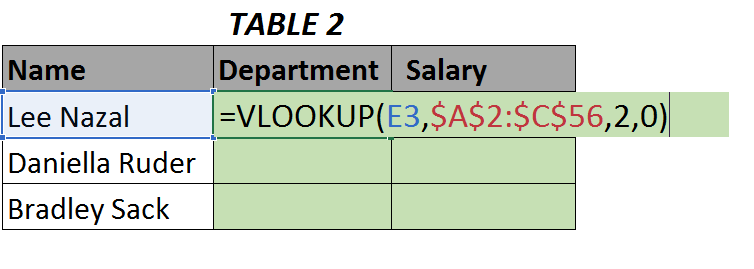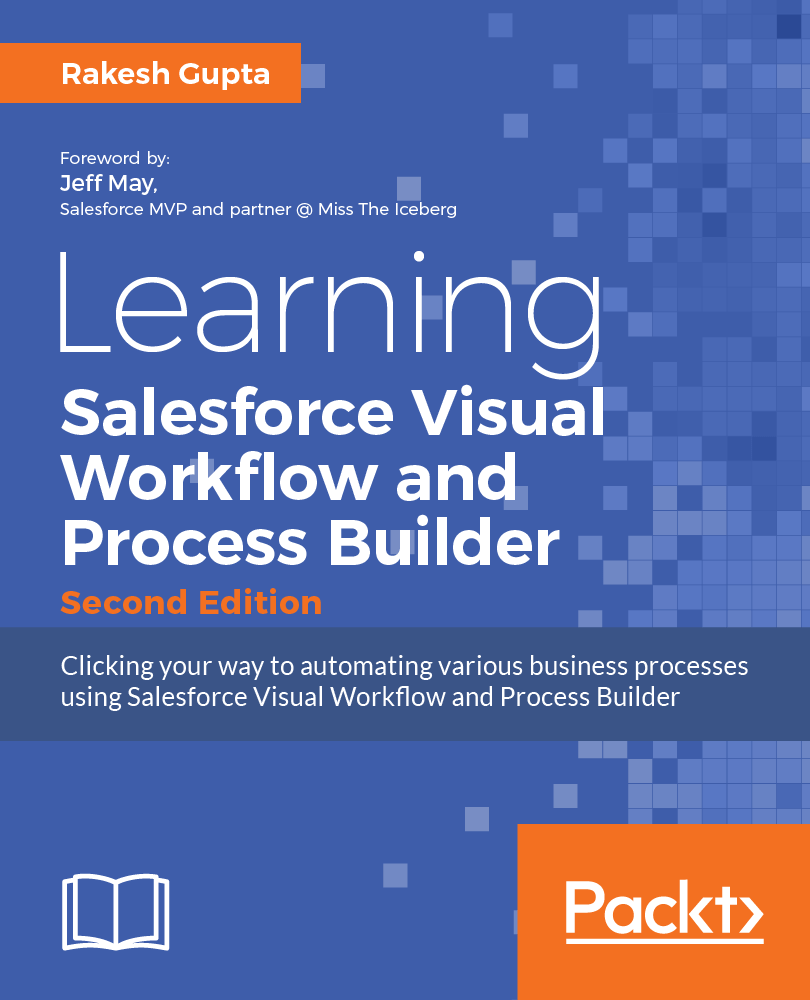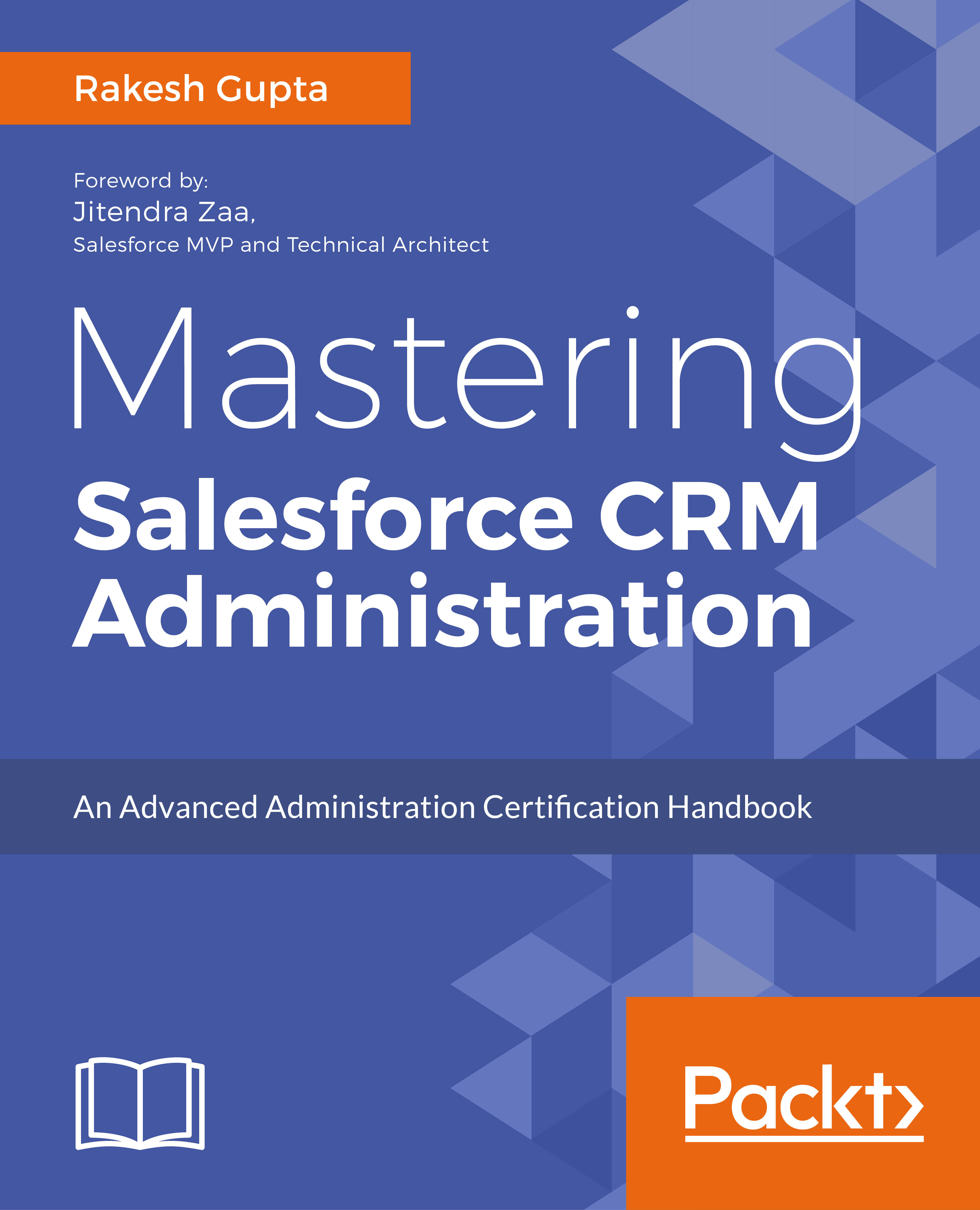• ## Quick-guide to VLOOKUP for automation experts

##### Posted at 8:26 PM by Rakesh Gupta, on July 6, 2017

*This post was contributed by guest author Hannah Sharron

VLOOKUP is one of the most well-known Excel functions – and not without reason!If I had to tell the most used function in excel, I would say VLOOKUPMany people would even say, that you have not learned Excel if you can’t perform a simple (V)LOOKUP. So, let us see what VLOOKUP is all about.

VLOOKUP has a super high versatile usage. This is a great thing, but many people find it hard to implement the function in practice.

VLOOKUP’s ease of use and simplicity when looking up data is unparalleled in Excel.

• Maybe you want to learn the basics of VLOOKUP step-by-step
• Perhaps your VLOOKUP formula isn’t working?

If yes, then you’ve come to the right place! Therefore, I’m going to give you a quick rundown on how to use VLOOKUP in a simple way.

Let’s look at an example:

How would you fill in data in the green cell in Table 2 using data from Table 1?## The Logic

Well, one simple approach is to use VLOOKUP. To do that let us first understand this function.

VLOOKUP or ‘Vertical Lookup’ helps in ‘looking up in vertical direction’ (hence the name VLOOKUP) a value in a data array or data organized in rows and columns.

Once it locates the ‘look-up-value’, corresponding column values can be pulled out.

So, if we wish to fill the green cells in Table 2 with a name (say Lee Nazal), we start by asking VLOOKUP to look for the name ‘Lee Nazal’ in Table 1 (which is row 9). Then VLOOKUP is to give us the corresponding value in the columns “Department” (which is the second column in Table 1) and “Salary” (which is column 3).

This is essentially how the logic of VLOOKUP works.

## Formula

Now we know the logic, here is how the formula looks like (also called its syntax):VLOOKUP is made up of 4 important fields, namely:

1. lookup_value: The value you want to look up in a data array and find its corresponding column values (i.e. cell E3).
2. table_array: This is the table where you want to pull out the data in Table 1 (i.e. \$A\$2:\$C\$56) – note the \$ sign.
3. col_index_num: This is important! So, in the example above we are looking for a Table 2 field – ‘Name’ in Table 1 and pulling out the corresponding Department and Salary. So, let’s see what the column numbers for ‘Department’ and ‘Salary’ in Table 1 are compared to look-up column (i.e. ‘Name’). Here, it’s easy to see that Department is ‘second’ and Salary is ‘third’ column. Note ‘Name’ is the first column. These relative positions (First=1, Second=2, Third=3) are the col_index_num!
4. range_lookup: This implies whether we want to find an exact(0) or approximate match (1) (for more information about the exact and approximate match in VLOOKUP, read here).

So, this is how the final formula looks:The real power of VLOOKUP is unleashed when it’s used with ‘cell-referencing’ which is beyond the scope of this article. But to give you a taste-test, by using correct referencing, VLOOKUP can easily be dragged around the green area above to populate the formula very quickly.

Here is a screenshot that demonstrates this:As we can see by putting \$ (E\$3) we can easily drag the formula down as shown by the gray arrow.

Note this would not be possible if we use E3 instead of E\$3.

Now if you have understood VLOOKUP, try filling up the salary column using the same approach using the attached exercise file (please link to attached exercise file).#### Author: Rakesh Gupta

6x Salesforce MVP | Solution Architect | Author of 7 books at Packtpub Publishing and Apress | 4x Dreamforce Speaker | Salesforce Coach | Co-host of AutomationHour.com

### 2 thoughts on “Quick-guide to VLOOKUP for automation experts”

•#### Bailey Rudd

##### June 6, 2017 at 12:07 PM

HI Rakesh,

Greats tips here that every excel user should know! I know there is a VLOOKUP functionality within Salesforce, now how is that function used? Is it similar to excels function and if so, wouldn’t a cross-object merge field work the same?

• #### Rakesh Gupta

##### June 6, 2017 at 12:56 PM

VLOOKUP concepts are similar in Salesforce. You can use it to find a potential duplicate in an object or set case priority to High only when a case is open for more than 36hrs. Remember these points while using VLOOKUP

1) Currently, VLOOKUP only available on Custom Objects.
2) Currently, VLOOKUP only available in Validation Rules.
3) Currently, VLOOKUP can only be done on the Name fields.
4) The field to return must be an auto number, roll-up summary, lookup relationship, master-detail relationship, checkbox, date, date/time, email, number, percent, phone, picklist, text, text area, or URL field type.
5) The field on lookup object must be the Record Name field on a custom object.
6) The field on lookup object and lookup value must be the same data type.

This site uses Akismet to reduce spam. Learn how your comment data is processed.

• #### Upcoming Trainings